Operations with Decimals

 Problem Set 1 Problem Set 2 Problem Set 3Select a problem set using the buttons above, then use your mouse or tab key to select a question. Fill in the blank with the correct answer. When you have answered all of the questions, ask Charlie how you did.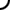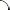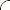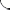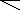Perform the indicated operation.   0.45 + 0.23 = 3 - 0.012 = 1.2(0.6) = 0.26 ÷ 13 = 0.008 + 0.007 = 25 ÷ 0.05 =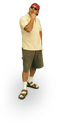Perform the indicated operation.   -2.56 + 3 = 0.03 - 0.5 = 8(0.3) = 0.16 ÷ 0.4 = 5 - 0.43 = 1.2(0.5) =Perform the indicated operation.   1.16 + 0.74 = 4 - 3.14 = 0.23(0.14) = 12 ÷ 0.06 = 0.113 + 0.494 = 0.0003(40) =©  2009  LarryPerezWeb Design:  Patrick Quigley